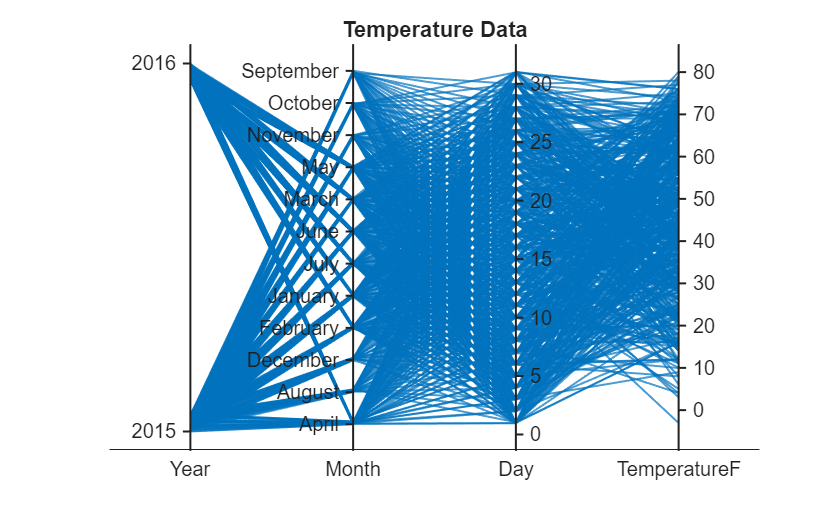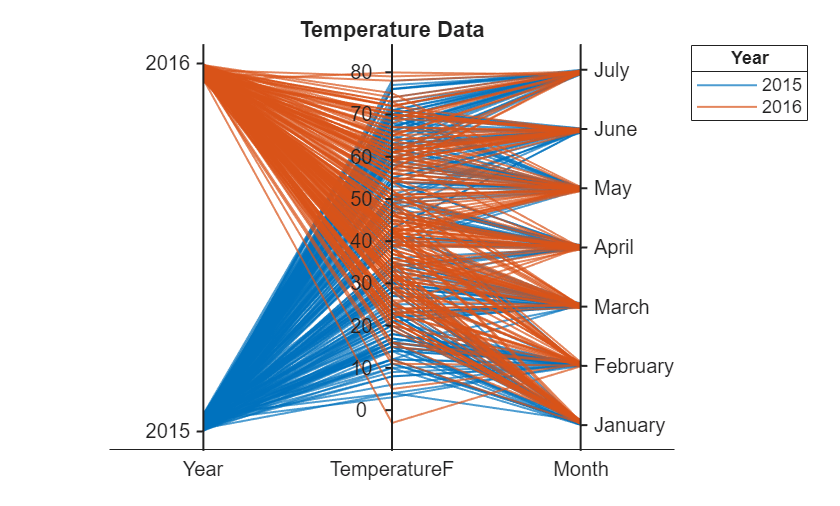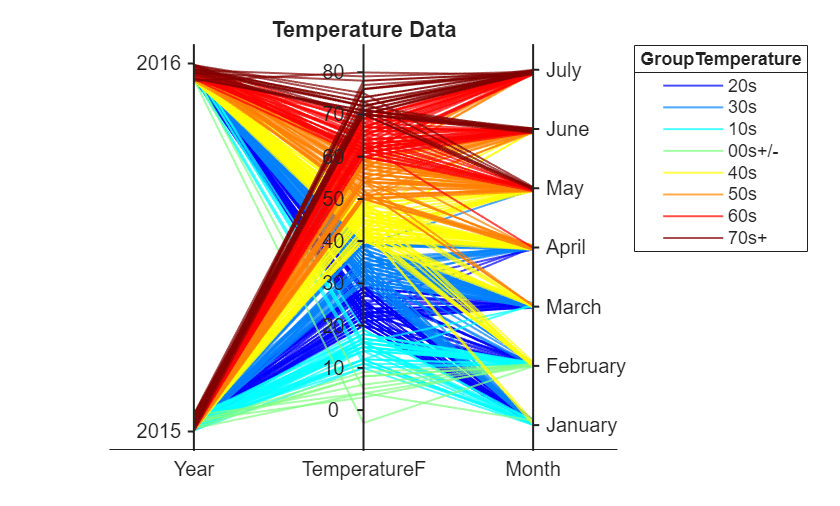# Explore Table Data Using Parallel Coordinates Plot

This example shows how to import a file into MATLAB® as a table, create a parallel coordinates plot from the tabular data, and modify the appearance of the plot.

Parallel coordinates plots are useful for visualizing tabular or matrix data with multiple columns. The rows of the input data correspond to lines in the plot, and the columns of the input data correspond to coordinates in the plot. You can group the lines in the plot to better see trends in your data.

### Import File as Table

Load the sample file `TemperatureData.csv`, which contains average daily temperatures from January 2015 through July 2016. Read the file into a table, and display the first few rows.

```tbl = readtable('TemperatureData.csv'); head(tbl)```
```ans=8×4 table Year Month Day TemperatureF ____ ___________ ___ ____________ 2015 {'January'} 1 23 2015 {'January'} 2 31 2015 {'January'} 3 25 2015 {'January'} 4 39 2015 {'January'} 5 29 2015 {'January'} 6 12 2015 {'January'} 7 10 2015 {'January'} 8 4 ```

### Create Basic Parallel Coordinates Plot

Create a parallel coordinates plot from the first few rows of the table. Each line in the plot corresponds to a single row in the table. By default, `parallelplot` displays all the coordinate variables in the table, in the same order as they appear in the table. The software displays the coordinate variable names below their corresponding coordinate rulers.

The plot shows that the first eight rows of the table provide temperature data for the first eight days in January 2015. For example, the eighth day was the coldest of the eight days, on average.

`parallelplot(head(tbl))`To help you interpret the plot, MATLAB randomly jitters plot lines by default so that they are unlikely to overlap perfectly along coordinate rulers. For example, although the first eight observations have the same `Year` and `Month` values, the plot lines are not flush with the `2015` tick mark along the `Year` coordinate ruler or the `January` tick mark along the `Month` coordinate ruler. Although jittering affects all coordinate variables, it is often more noticeable along categorical coordinate rulers because it depends on the distance between tick marks. You can control the amount of jittering in the plot by setting the `Jitter` property.

Notice that some of the tick marks along the `Year` coordinate ruler are meaningless decimal values. To ensure that tick marks along a coordinate ruler correspond only to meaningful values, convert the variable to a categorical variable by using the `categorical` function.

`tbl.Year = categorical(tbl.Year);`

Now create a parallel coordinates plot from the entire table. Assign the `ParallelCoordinatesPlot` object to the variable `p`, and use `p` to modify the plot after you create it. For example, add a title to the plot using the `Title` property.

`p = parallelplot(tbl)`
```p = ParallelCoordinatesPlot with properties: SourceTable: [565x4 table] CoordinateVariables: {'Year' 'Month' 'Day' 'TemperatureF'} GroupVariable: '' Show all properties ```
`p.Title = 'Temperature Data';`### Group Plot Lines

Group the lines in the plot according to the `Year` values by setting the `GroupVariable` property. By default, MATLAB adds a legend to the plot. You can remove the legend by setting the `LegendVisible` property to `'off'`.

`p.GroupVariable = 'Year';`### Rearrange Coordinate Variables Interactively

Rearrange coordinate variables interactively to compare them more easily and decide which variables to keep in your plot.

Open your plot in a figure window. Click a coordinate tick label and drag the associated coordinate ruler to the location of your choice. The software outlines the selected coordinate ruler in a black rectangle. For example, you can click the `Month` coordinate tick label and drag the coordinate ruler to the right. You can then easily compare `Month` and `TemperatureF` values.When you rearrange coordinate variables interactively, the software updates the associated `CoordinateTickLabels`, `CoordinateVariables`, and `CoordinateData` properties of the plot.

For more interactivity options, see Tips.

### Select Subset of Coordinate Variables

Display a subset of the coordinate variables in `p.SourceTable` and specify their order in the plot by setting the `CoordinateVariables` property of `p`.

In particular, remove the `Day` variable from the plot, and display the `TemperatureF` variable, which is in the fourth column of the source table, as the second coordinate in the plot.

`p.CoordinateVariables = [1 4 2];`Alternatively, you can set the `CoordinateVariables` property by using a string or cell array of variable names or a logical vector with `true` elements for the selected variables.

### Modify Categories in Coordinate Variable

Display a subset of the categories in `Month` and change the category order along the coordinate ruler in the plot.

Because some months have data for only one of the two years, remove the rows in the source table corresponding to those unique months. MATLAB updates the plot as soon as you change the source table.

```uniqueMonth = {'September','October','November','December','August'}; uniqueMonthIdx = ismember(p.SourceTable.Month,uniqueMonth); p.SourceTable(uniqueMonthIdx,:) = [];```Arrange the months in chronological order along the `Month` coordinate ruler by updating the source table.

```categoricalMonth = categorical(p.SourceTable.Month); newOrder = {'January','February','March','April','May','June','July'}; orderMonth = reordercats(categoricalMonth,newOrder); p.SourceTable.Month = orderMonth;```### Group Plot Lines Using Binned Values

To better visualize the range of temperatures during each month, bin the temperature data by using `discretize` and group the lines in the plot using the binned values. Check the minimum and maximum temperatures in the source table. Set the bin edges such that they include these values.

`min(p.SourceTable.TemperatureF)`
```ans = -3 ```
`max(p.SourceTable.TemperatureF)`
```ans = 80 ```
```binEdges = [-3 10:10:80]; bins = {'00s+/-','10s','20s','30s','40s','50s','60s','70s+'}; groupTemperature = discretize(p.SourceTable.TemperatureF,binEdges,'categorical',bins);```

Add the binned temperatures to the source table. Group the lines in the plot according to the binned temperature data.

```p.SourceTable.GroupTemperature = groupTemperature; p.GroupVariable = 'GroupTemperature';```Because `GroupTemperature` includes more than seven categories, some of the groups have the same color in the plot. Assign distinct colors to every group by setting the `Color` property.

`p.Color = jet(8);`